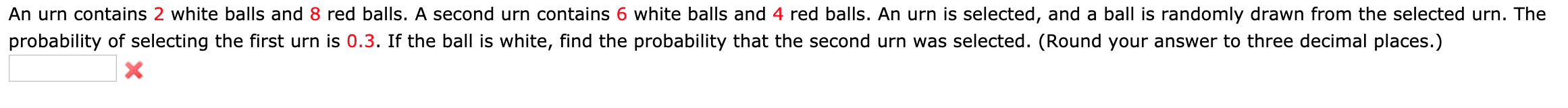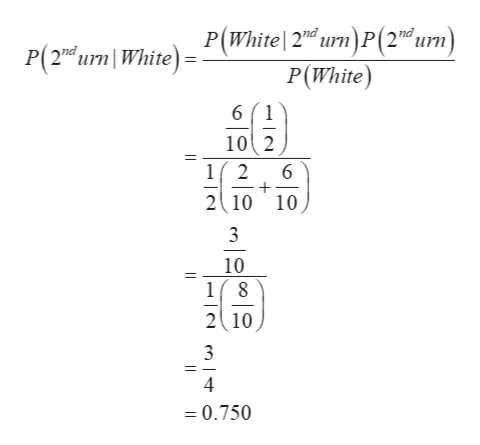An urn contains 2 white balls and 8 red balls. A second urn contains 6 white balls and 4 red balls. An urn is selected, and a ball is randomly drawn from the selected urn. Theprobability of selecting the first urn is 0.3. If the ball is white, find the probability that the second urn was selected. (Round your answer to three decimal places.)X

Questionhelp_outlineImage TranscriptioncloseAn urn contains 2 white balls and 8 red balls. A second urn contains 6 white balls and 4 red balls. An urn is selected, and a ball is randomly drawn from the selected urn. The probability of selecting the first urn is 0.3. If the ball is white, find the probability that the second urn was selected. (Round your answer to three decimal places.) X fullscreen
Step 1

If the ball is white, the probability that the s...help_outlineImage TranscriptioncloseP(2n urn WhiteP(White|2nd urn)P(2d urn) P(White) 61 102 1(2 2 10 10 6 - 3 10 18 2 10 - 3 4 0.750 fullscreen

Want to see the full answer?

See Solution

Want to see this answer and more?

Our solutions are written by experts, many with advanced degrees, and available 24/7

See Solution
Tagged in

Basic Probability